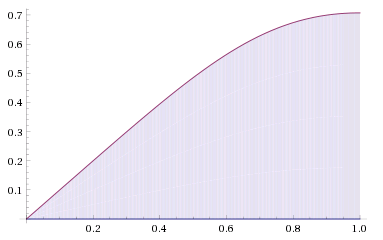# To The End of The Line

Calculus Level 4$\displaystyle \lim_{n \to \infty} \sum_{k=1}^n \frac {k}{\sqrt{n^4+k^4}} = \frac {a}{d} \ \ln \left ( \sqrt b + c \right )$

The above summation is fulfilled for positive integers $a,b,c,d$, with $a,d$ coprime and $b$ square-free.

What is the value of $a+b+c+d$?

×